# Weight and Mass

People often talk about weight and mass as if they are the same thing, but are they? The mass of an object is the amount of matter that it is made up of, so it never changes (unless you take some of the matter away). An object's weight can change, depending on where you measure it. Let's find out more.

## Mass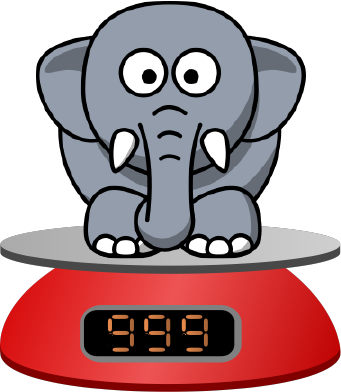This is a young elephant, called Eric. If we pop him on the scales, they tell us that his mass is $999$ kg. Mass is the amount of matter in an object. It is measured in kilograms (kg). An object's mass never changes.

## What is weight?The scales actually measure Eric's weight and convert it into the mass that they report. The weight of an object measures the force that gravity places on it. Gravity is pretty much the same, no matter where you go on Earth, so these scales (designed on Earth) can easily convert from weight to mass. They simply dividing by a fixed number called Earth's gravitational constant. This fixed number is about $9.8$ on Earth. There may be slight differences in the mass your scales report at different places on Earth, but they won't be very large.

Weight is a force that is measured in Newtons. It is the pull that is placed on an object because of gravity. One Newton is one kilogram metre per second squared, and is abbreviated as $N$.

On Earth, gravity exerts a pull of approximately 9.8 N on a 1 kilogram mass. So, Eric really weighs about $999 \times 9.8 = 9,790$ N on Earth. If we take Eric somewhere else, where the pull caused by gravity is weaker or stronger, then Eric's weight will change. His mass will stay the same.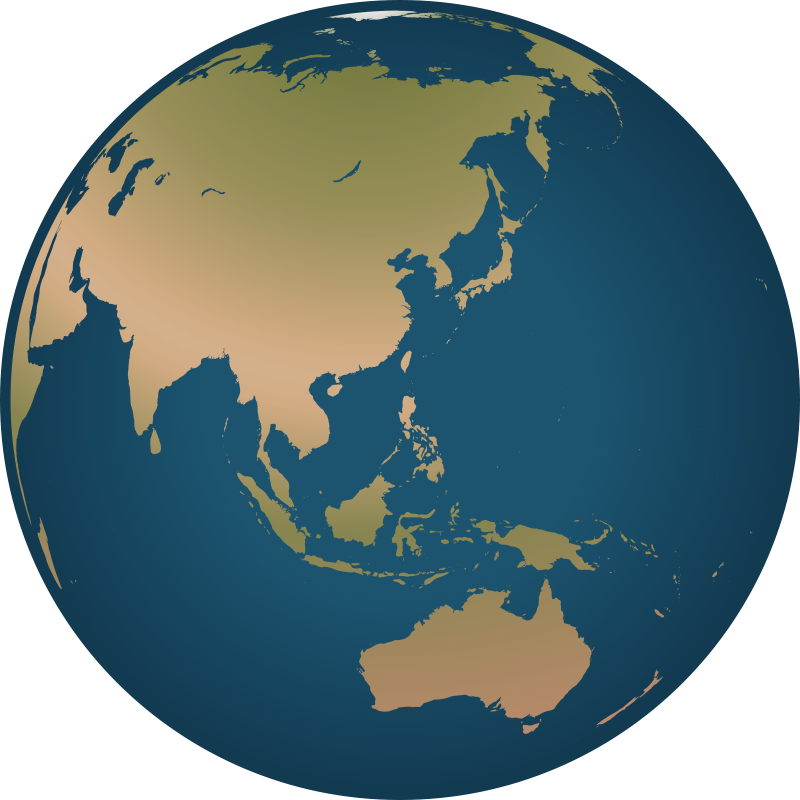Let's take Eric on a trip to visit some other parts of our Solar System. We'll take the scales with us as well, and see what they read

### Elephants in Space

We're going to use our scales to get an idea of how Eric's weight changes as we move to different parts of the solar system. Remember, Eric's mass will stay the same, but because the scales are expecting the pull of gravity to be the same as the pull of gravity on Earth, their readings will be different.

#### In Outer Space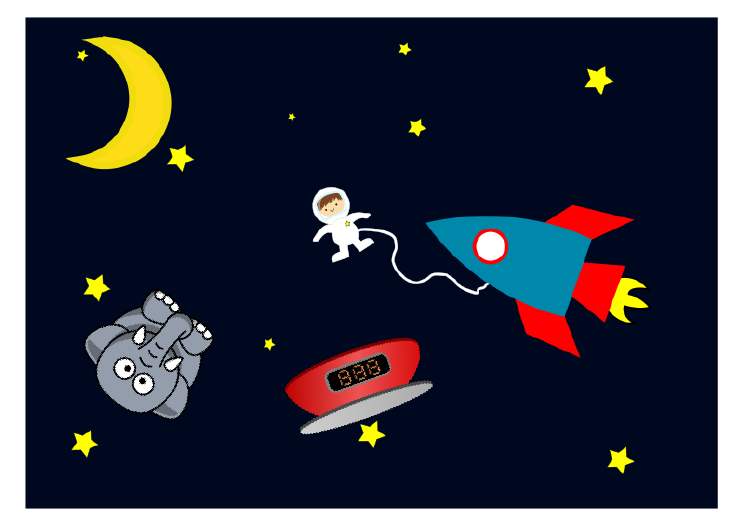Eric and Christo decide to go for a space walk. Elephants aren't very good at gymnastics on Earth because they weigh too much, but look at what Eric can get up to out here! That was a very graceful cartwheel, Eric. Well, done!

There is almost no force due to gravity in outer space, so if Eric was able to stand on the scales, they'd read $0$ kg. Remember, his mass is still $999$ kg, but the scales don't register that because they really report his weight, which would be $0$ N out here.

#### On the Moon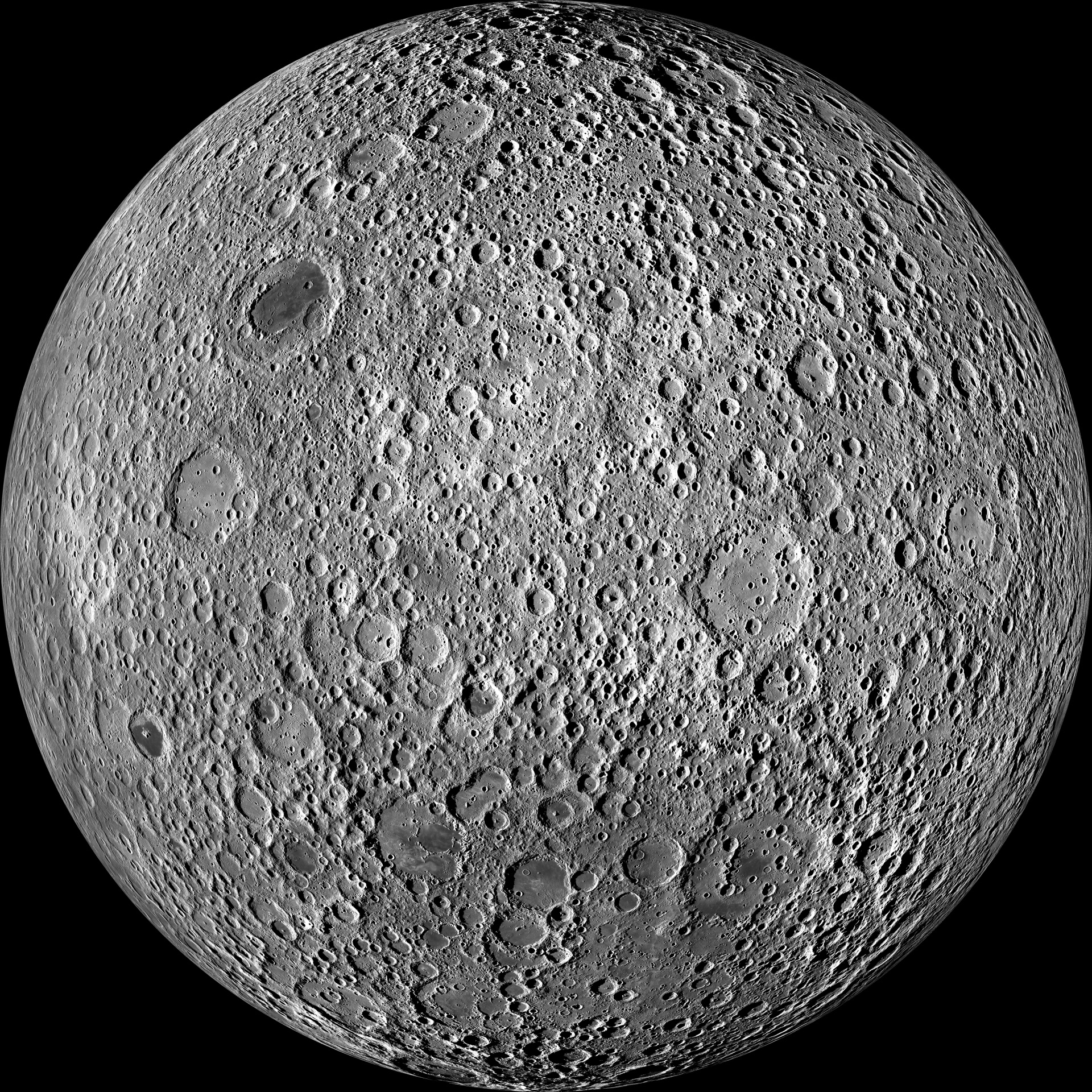Did you know that elephants can't jump? Part of the reason for this is that their feet aren't strong enough, and they weigh so much. The pull of gravity on the moon is only about $\dfrac{1}{6}$ as much as on the Earth. Eric's weight on the moon is about $1598 \text{ N}$, so the scales will report $163$ kg. Remember, his mass hasn't changed at all, this is just because of the smaller pull of gravity. Can you hear Eric? "Wheeeeeeeeee!" He's having a great time. Is it any wonder that Eric can bounce about like this on the moon?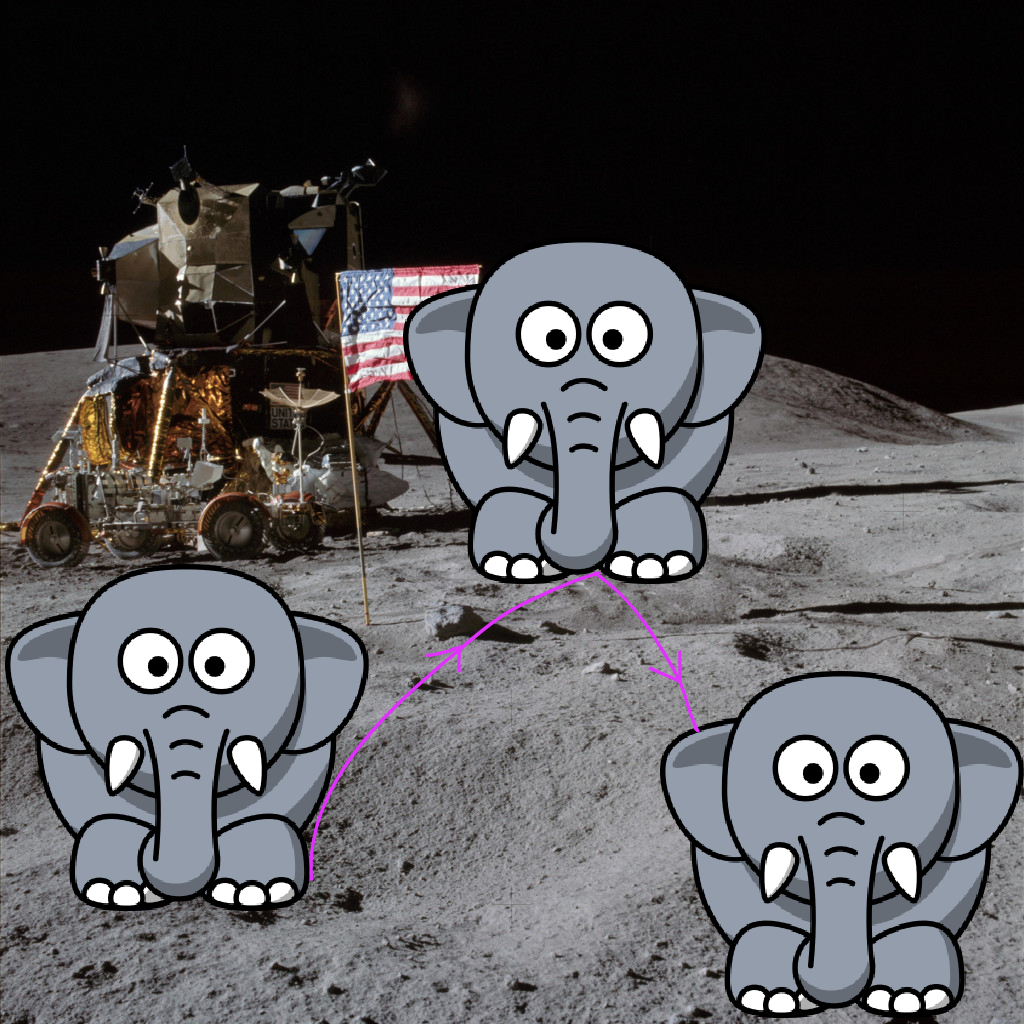#### On Jupiter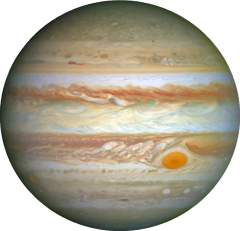Jupiter is a much heavier planet than the Earth, so the pull exerted by gravity on Jupiter is much bigger than on the Earth. In fact, Eric's weight is $24,775$ N on Jupiter. Unfortunately, we can't get a read out from the scales, because this heavy weight has broken them, but the scales would read $2,528$ kg if they could!

Just remember, Eric's mass hasn't changed. The pull of gravity has become a lot stronger, so his weight has become much larger.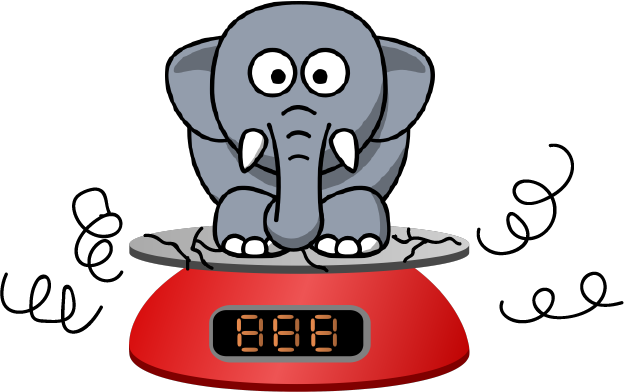### Apparent Weight

An apparent measures a downwards force on an object. It is not the same as a real weight, because the force of gravity has not changed, but you can increase or decrease the downwards force that acts on an object.

You can trick the scales into thinking you weigh more or less on Earth. There are a few things that can change the reading. Remember, your mass hasn't changed, it's just that the scales have been fooled.

Here are some experiments you can try to see how your scales can be tricked. However, your parents might not be too impressed if they catch you jumping up and down on the scales.

• Change the surface you weigh yourself on: do you appear to be heavier if you put the scales on carpet or if you put them on the bathroom tiles?
• Jump up and down on the scales (gently). What happens to the reading on the scales?
• Weigh yourself in a lift. Do you weigh more when the lift is moving upwards, or when the lift is moving downwards?

## Conclusions

Weight and mass are two different, but related things. A mass tells you how much matter is in an object, while a weight tells you how strongly gravity pulls on an object.

The apparent weight of an object tells you what downwards force acts on the object. This can be because of a combination of the action of gravity and other forces.

Weight is a force, so it is measured in Newtons.

Scales give you an estimate of your mass. On Earth, they divide your weight by $9.8$ to give this estimate.

### Description

This mini book covers the core of Math for Foundation, Grade 1 and Grade 2 mathematics including

1. Numbers
3. Subtraction
4. Division
5. Algebra
6. Geometry
7. Data
8. Estimation
9. Probability/Chance
10. Measurement
11. Time
12. Money
13. and much more

This material is provided free of cost for Parent looking for some tricks for their Prekinder, Kinder, Prep, Year 1 and Year 2 children

### Learning Objectives

These lessons are for kids aged 4-8 with the core objective to expose their brains to concepts of addition, subtraction, division, algebra and much more.

Author: Subject Coach
You must be logged in as Student to ask a Question.